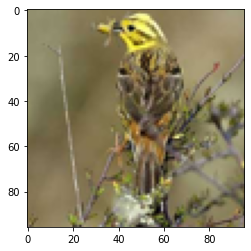Learn data science and machine learning by building real-world projects on Jovian

## Classifying STL10 images of everyday objects using neural network, CNN, ResNets, Regularization and Data Augmentation in PyTorch

A.K.A. Training an image classifier from scratch to over 90% accuracy in less than 5 minutes on a single GPU

#### Part of Course project of "Deep Learning with Pytorch: Zero to GANs"

The ability to try many different neural network architectures to address a problem is what makes deep learning really powerful, especially compared to shallow learning techniques like linear regression, logistic regression etc.

In this notebook we will:

1. Explore the STL10 dataset: https://cs.stanford.edu/~acoates/stl10/
2. Set up a training pipeline to train a neural network on a GPU
3. Experiment with different network architectures & hyperparameters
In :
``````# Uncomment and run the commands below if imports fail
# !conda install numpy pandas pytorch torchvision cpuonly -c pytorch -y
# !pip install matplotlib --upgrade --quiet``````

Installing and Importing the required modules and classes from torch, torchvision, numpy, and matplotlib.

In :
``````import os
import torch
import  torchvision
import numpy as np
import matplotlib.pyplot as plt
import torch.nn as nn
import torch.nn.functional as F
from torchvision.datasets import STL10
from torchvision.transforms import ToTensor
from torchvision.utils import make_grid
from torch.utils.data import random_split
%matplotlib inline
``````
In :
``````# Project name used for jovian.commit
project_name = '06-stl10-project'``````
In :
``````from google.colab import drive
drive.mount('/content/drive')``````
```Mounted at /content/drive ```

### Exploring the STL10 dataset

We download the data and create a PyTorch dataset using the STL10 class from torchvision.datasets.

In :
``````# path to store/load data
test_dataset = STL10(root='/content/drive/MyDrive/data', split ='test', transform=ToTensor())
#test_dataset = STL10(root='data/', split ='test', transform=ToTensor())``````
```Files already downloaded and verified ```

The No of images in the training and testing dataset List the no of output classes in the dataset

In :
``````dataset_size = len(dataset)
train_ds = dataset
test_dataset_size = len(test_dataset)
classes = dataset.classes
dataset_size,train_ds.data.shape,test_dataset_size,dataset.classes,len(dataset.classes)``````
Out:
``````(5000,
(5000, 3, 96, 96),
8000,
['airplane',
'bird',
'car',
'cat',
'deer',
'dog',
'horse',
'monkey',
'ship',
'truck'],
10)``````

The shape of an image tensor from the dataset

In :
``````img, label = train_ds
img_shape = img.shape
img_shape
``````
Out:
``torch.Size([3, 96, 96])``

Note that this dataset consists of 3-channel color images (RGB). Let us look at a sample image from the dataset. `matplotlib` expects channels to be the last dimension of the image tensors (whereas in PyTorch they are the first dimension), so we'll the `.permute` tensor method to shift channels to the last dimension. Let's also print the label for the image.

In :
``````img, label = dataset
plt.imshow(img.permute((1, 2, 0)))
print('Label (numeric):', label)
print('Label (textual):', classes[label])``````
```Label (numeric): 1 Label (textual): bird ```The number of images belonging to each class

In :
``````count_class = {}
for _,outs in dataset:
labels = classes[outs]
if labels not in count_class:
count_class[labels] = 0
count_class[labels] += 1
count_class
``````
Out:
``````{'airplane': 500,
'bird': 500,
'car': 500,
'cat': 500,
'deer': 500,
'dog': 500,
'horse': 500,
'monkey': 500,
'ship': 500,
'truck': 500}``````

Let's save our work to Jovian, before continuing.

In :
``!pip install jovian --upgrade --quiet``
In :
``import jovian``
In :
``jovian.commit(project=project_name, environment=None)``
```[jovian] Detected Colab notebook... [jovian] Please enter your API key ( from https://jovian.ai/ ): API KEY: ·········· [jovian] Uploading colab notebook to Jovian... [jovian] Committed successfully! https://jovian.ai/venkatesh-vran/06-stl10-project ```
Out:
``'https://jovian.ai/venkatesh-vran/06-stl10-project'``
###### Preparing the data for training

We'll use a validation set with 1500 images . To ensure we get the same validation set each time, we'll set PyTorch's random number generator to a seed value of 43.

In :
``````torch.manual_seed(43)
val_size = 1500
test_size = len(test_dataset) - val_size``````

Let's use the `random_split` method to create the training & validation sets

In :
``````test_ds, val_ds = random_split(test_dataset, [test_size, val_size])
len(test_ds), len(val_ds)``````
Out:
``(6500, 1500)``

We can now create data loaders to load the data in batches.

In :
``batch_size=128``
In :
``````train_loader = DataLoader(train_ds, batch_size, shuffle=True, num_workers=4, pin_memory=True)
Let's visualize a batch of data using the `make_grid` helper function from Torchvision.
``````for images, _ in train_loader:
```images.shape: torch.Size([128, 3, 96, 96]) ```